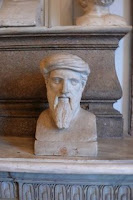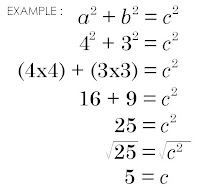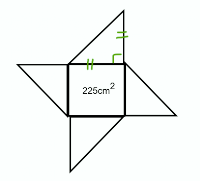### Cure For Harbeck's Amnesia

Sunday, February 22, 2009
Mr. Harbeck supposedly slipped on some ice, fell and bumped his head which caused him to forget what he was going to teach us. To help him regain his memory, we were assigned:

• To explain the four artifacts
• How These Words Relate: Legs, hypotenuse, R.A.T., Greek, and theorem.

Artifact #1:The first artifact is known as a right triangle or a R.A.T. (right angle triangle). What makes this triangle a R.A.T. / right triangle, is the 90° right angle where the square on the bottom left corner is placed. The two sides that create this right angle are called legs. The other two angles are called theta and beta, together they equal to 90°, which makes them complementary angles. The longest side of the triangle or the side across from the right angle, is known as the hypotenuse. The hypotenuse is usually labelled as "C" and the legs are labelled as "A" and "B".

Artifact #2:The second artifact is a square. The lines on each side of the square, are called lines of symmetry. This shows all the sides are equal to each other, making it a square and not a rectangle. In each of the four corners, are 90° right angles. Together, the whole square is 360° (4 x 90° = 360°). If you were to cut this square diagonally in half, you'd have two right triangles, like in the first artifact.

Artifact #3:Who is this a sculpture of? Well he is a Greek man of brilliance. He is thought to be the 'Father of Math'. This man's name is Pythagoras and was a vegan (someone whom doesn't consume any animal products). He was a mathematician who loved music. Pythagoras was the first to figure out the circumference of the earth and proved that the earth revolves around the sun. He was very fluent in Egyptian and was interested in harmonics. He also invented the Pythagorean Theorem, a² + b² = c².The Pythagorean Theorem is a formula or theory that can be proved. This theorem finds out the length of the side 'C' on a right triangle. To figure out the length of 'C', you need to know what sides 'A' and 'B' are. Next, you square 'A' and 'B' and add them together, this will leave you with 'C²'. Last but not least, you must find the square root of the number and that gives you the length of 'C'.

Pythagorean Theorem Video Part One - Krissia, Carrie and I

Pythagorean Theorem Part Two - Krissia, Carrie and I

Problem#1:In this problem that Mr.Harbeck gave us, we had to find the base of the triangle. This picture explains how I solved 'C' using the Pythagorean Theorem.

Problem #2The first you need to do is, you need to find out each side of the square. Then that will give you sides 'A' and 'B' for all the triangles, since they're all the same length. Since you know sides 'A' and 'B', you need to find the length for the hypotenuse, which is 'c'. Once you have the length of 'C', add up all the sides together which will give you the perimeter.